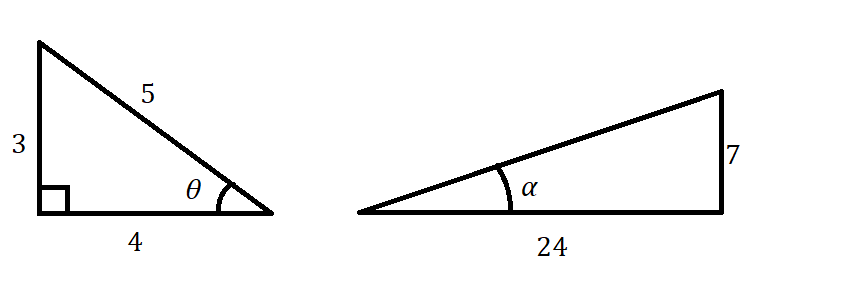# Find the exact value of sin 60°.Question
Trigonometric FunctionsFind the exact value of sin 60°.2021-03-02
Use the following trivial identity: $$\displaystyle{\sin{{\left({60}°\right)}}}=\frac{\sqrt{{3}}}{{2}}$$
Decimal: 0.86602...

### Relevant QuestionsThe question asks for the exact value of the trigonometric function at the given real number:
$$\displaystyle{\sin{{\left(\frac{{{3}\pi}}{{4}}\right)}}}$$Use the figures to find the exact value of the trigonometric function $$\displaystyle{\tan{{2}}}\theta$$.sec theta = -3, tan theta > 0ZSK. Find the exact value of the remaining trigonometric functions of
thetaZSK.Find the exact value of the trigonometric function $$\displaystyle{\sec{{\left(\frac{{-{9}\pi}}{{4}}\right)}}}$$ .Find the exact value of the trigonometric function $$\displaystyle\frac{{\cos{{\left({9}\pi\right)}}}}{{4}}$$.Use a calculator to find the value of the trigonometric function $$\displaystyle{\sin{{\left(\frac{{{3}\pi}}{{10}}\right)}}}$$ to four decimal places.$$\displaystyle{y}={\sin{{\left(\pi{x}\right)}}}$$$$\displaystyle{\sin{{t}}}=\frac{{3}}{{4}}{\quad\text{and}\quad}{\cos{{t}}}=\frac{\sqrt{{7}}}{{4}}$$Find the values of the other trigonometric functions of theta if $$\displaystyle{\cot{\theta}}=-\frac{{4}}{{3}}{\quad\text{and}\quad}{\sin{\theta}}{<}{0}$$.Find derivative of trigonometric function $$\displaystyle{y}=\frac{{{3}{\left({1}-{\sin{{x}}}\right)}}}{{{2}{\cos{{x}}}}}$$